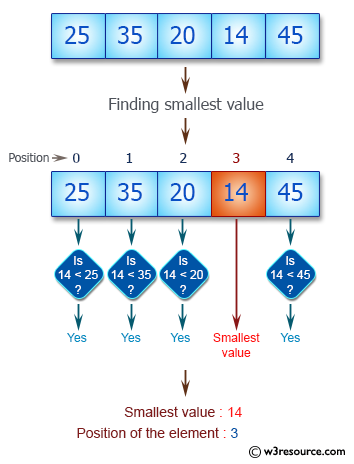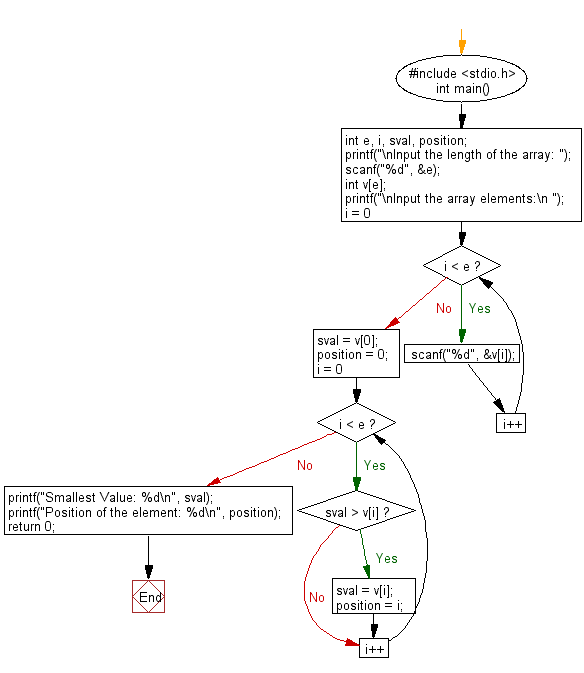﻿ C : Find the smallest element and its position in an array

# C Exercises: Find the smallest element and its position in an array

## C Basic Declarations and Expressions: Exercise-52 with Solution

Write a C program to read an array of length 6 and find the smallest element and its position.

Pictorial Presentation:Sample Solution:

C Code:

``````#include <stdio.h>
int main() {
int e, i, sval, position;

// Prompt user to input the length of the array
printf("\nInput the length of the array: ");
scanf("%d", &e);

// Declare an array of length 'e'
int v[e];

// Prompt user to input the array elements
printf("\nInput the array elements:\n ");
for(i = 0; i < e; i++) {
scanf("%d", &v[i]);
}

// Initialize 'sval' and 'position' with the first element of the array
sval = v;
position = 0;

// Find the smallest value and its position in the array
for(i = 0; i < e; i++) {
if(sval > v[i]) {
sval = v[i];
position = i;
}
}

// Print the smallest value and its position
printf("Smallest Value: %d\n", sval);
printf("Position of the element: %d\n", position);

return 0;
}
``````

Sample Output:

```Input the length of the array: 5

Input the array elements:
25
35
20
14
45
Smallest Value: 14
Position of the element: 3
```

Flowchart:C programming Code Editor:

What is the difficulty level of this exercise?

Test your Programming skills with w3resource's quiz.

﻿